# MCAT Physical : Friction and Normal Force

## Example Questions

← Previous 1

### Example Question #1 : Forces

A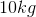object is originally at rest on an inclined plane, which forms an angle with the ground of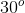. If the coefficient of static friction is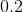, what is the force of friction that must be overcome for the object to begin moving?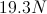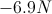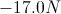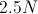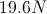Explanation:

In this situation, we must simply remember how to calculate friction. Once the force of gravity overcomes the force of static friction, the object will slide. Our formula for friction is: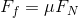This means the force of friction is equal to the friction coefficient times the normal force. On an incline, the normal force is equal to the force of gravity times the cosine of the angle: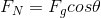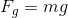We can combine our formulas to give the force of friction.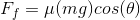Using the given coefficient of friction, mass, and angle, we can calculate the force of friction.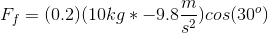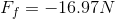### Example Question #2 : Forces

When the force applied to a moving object is equal and opposite the force of kinetic friction, what happens to the object?

It moves with a constant velocity

It decelerates

It accelerates

It changes direction

It stops

It moves with a constant velocity

Explanation:

It is important to understand the difference between static and kinetic friction. When an object is at rest, it takes more force to get it to start moving than to keep it moving. If you match the amount of static friction that can be generated when the object is at rest, it will not move because there is zero net force; the force applied must be greater than the static friction in order to initiate motion. Once the object begins moving, the force required to keep it moving decreases. If you match the force of kinetic friction, the object moves at a constant velocity because there is again no net force. Any more force will cause acceleration, while any less will cause deceleration.

### Example Question #3 : Forces

A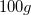block rests on a wooden table. A spring scale attached to the right side of the block is very gently pulled to the right with increasing force. Which of these is true, assuming a frictional force between the table and the block?

The block will not move until the spring scale reads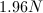The block will move to the right proportional to the force applied to it

The block will move to the right at a uniform velocity

When the block begins to move, it will smoothly accelerate to the right

The block will suddenly jerk to the right, and then stop

The block will suddenly jerk to the right, and then stop

Explanation:

This is a classic experiment to determine the coefficient of static friction,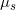. The frictional interaction of the block on the table causes an equal and opposite force to be generated against the pulling on the spring scale. The coefficient of static friction represents the ratio of the horizontal forces caused by surface irregularities to the vertical force due to gravity.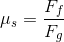When the frictional force is just barely overcome, the block will start moving to the right, but as soon as it does, the tension in the spring diminishes and static frictional forces prevail. The block thus starts moving and almost immediately stops.

### Example Question #4 : Forces

Ablock rests on a wooden table. What is the force of the table upon the block?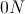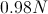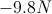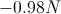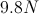Explanation:

In this question, the net force on the block is zero. We know from Newton's second law that any non-zero force will produce an acceleration, resulting in movement of some sort. Since the block is at rest, and not moving, we can conclude that the net force is zero.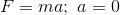The forces acting on the block are the force of gravity, pulling the block downward, and the normal force, pushing the block upward. The force of the block on the table will be the force from gravity, while the force of the table on the block will be the normal force. Since these are the only two forces acting on the block, we can add them together to get the net force.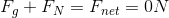Reorganizing the equation, we can set the two forces equal. This is a reflection of Newton's third law.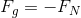Gravitational force is equal to the mass of the object times the acceleration from gravity.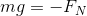Using these values, given in the question, we can find the normal force, or the force of the table on the block.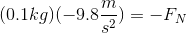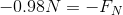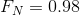The final normal force is positive because it acts in the upward direction, opposite of gravity.

### Example Question #5 : Forces

Two children are playing with sleds on a snow-covered hill. Sam weighs 50kg, and his sled weighs 10kg. Sally weighs 40kg, and her sled weighs 12kg. When they arrive, they climb up the hill using boots. Halfway up the 50-meter hill, Sally slips and rolls back down to the bottom. Sam continues climbing, and eventually Sally joins him at the top.

They then decide to sled down the hill, but disagree about who will go first.

Scenario 1:

Sam goes down the hill first, claiming that he will reach a higher velocity.  If Sally had gone first, Sam says they could collide.

Scenario 2:

Sally goes down the hill first, claiming that she will experience lower friction and thus reach a higher velocity. If Sam had gone first, Sally says they could collide.

Scenario 3:

Unable to agree, Sam and Sally tether themselves with a rope and go down together.

Who would you expect to experience a greater force of friction while traveling down the hill?

They would experience equal forces of friction because the coeffecients of friction are the same for both.

Sally, because she travels more slowly

Sam, because he experiences a greater normal force

Sally, because she experiences less normal force

Sam, because he travels more quickly

Sam, because he experiences a greater normal force

Explanation:

The force of friction can be found from the following equation.

Force of Friction = Normal Force * Coeffecient of Friction

Normal force here is the force that the Earth pushes back on Sam against his mass. Thus, because he is more massive, he will experience a greater normal force and greater frictional force as a result.

### Example Question #6 : Forces

Two children are playing with sleds on a snow-covered hill. Sam weighs 50kg, and his sled weighs 10kg. Sally weighs 40kg, and her sled weighs 12kg. When they arrive, they climb up the hill using boots. Halfway up the 50-meter hill, Sally slips and rolls back down to the bottom. Sam continues climbing, and eventually Sally joins him at the top.

They then decide to sled down the hill, but disagree about who will go first.

Scenario 1:

Sam goes down the hill first, claiming that he will reach a higher velocity. If Sally had gone first, Sam says they could collide.

Scenario 2:

Sally goes down the hill first, claiming that she will experience lower friction and thus reach a higher velocity. If Sam had gone first, Sally says they could collide.

Scenario 3:

Unable to agree, Sam and Sally tether themselves with a rope and go down together.

When the force of friction acts on a moving body, as it would on Sam when moving down the hill, __________.

Entropy of the universe decreases because movement decreases as Sam slows to a stop

Friction always acts opposite the direction of movement

Entropy increases in the universe

Friction can be totally recaptured and used to power future movements

Friction is inversely proportional to the normal force

Entropy increases in the universe

Explanation:

This is a tricky question. Entropy of the universe increases because, even though Sam's movement does slow down resulting in a local decline in entropy, the heat generated by friction with the ground results in a net increase in entropy overall.

Another tempting choice would be that friction always acts opposite the direction of motion. Friction always acts opposite to the direction of RELATIVE motion, not necessarily to the direction of motion in which the body itself is traveling.

### Example Question #7 : Forces

Two children are playing with sleds on a snow-covered hill. Sam weighs 50kg, and his sled weighs 10kg. Sally weighs 40kg, and her sled weighs 12kg. When they arrive, they climb up the hill using boots. Halfway up the 50-meter hill, Sally slips and rolls back down to the bottom. Sam continues climbing, and eventually Sally joins him at the top.

They then decide to sled down the hill, but disagree about who will go first.

Scenario 1:

Sam goes down the hill first, claiming that he will reach a higher velocity. If Sally had gone first, Sam says they could collide.

Scenario 2:

Sally goes down the hill first, claiming that she will experience lower friction and thus reach a higher velocity. If Sam had gone first, Sally says they could collide.

Scenario 3:

Unable to agree, Sam and Sally tether themselves with a rope and go down together.

What would you expect is true of Sam before he starts moving?

There would be more friction acting on Sam while he moved down the hill if he weighed less, since he would be traveling more slowly

Friction can only act on a body while it is slowing down

The maximum force of friction acting on Sam is less before Sam starts moving than once he is sliding down the hill

There can only be friction acting on Sam when he is in motion

The maximum force of friction acting on Sam is greater before Sam starts moving than once he is sliding down the hill

The maximum force of friction acting on Sam is greater before Sam starts moving than once he is sliding down the hill

Explanation:

Static friction exerts a greater effect than does kinetic friction. Think about if you are trying to slide a heavy box across a room. It takes some force to get it going, but once it is moving it takes comparatively less force to keep it moving

### Example Question #8 : Forces

Two children are playing on an icy lake. Child 1 weighs 50kg, and child 2 weighs 38kg. Child 1 has a backpack that weighs 10kg, and child 2 has a backpack that weighs 5kg.

Over the course of the afternoon, they collide many times. Four collisions are described below.

Collision 1:

Child 1 starts from the top of a ramp, and after going down, reaches the lake surface while going 5m/s and subsequently slides into a stationary child 2. They remain linked together after the collision.

Collision 2:

Child 1 and child 2 are sliding in the same direction. Child 2, moving at 10m/s, slides into child 1, moving at 2m/s.

Collision 3:

The two children collide while traveling in opposite directions at 10m/s each.

Collision 4:

The two children push off from one another’s back, and begin moving in exactly opposite directions. Child 2 moves with a velocity of +8m/s.

Imagine that the above collisions happened on another planet, where the gravitational constant was exactly half that of Earth. Compared to the force of friction acting on moving bodies on Earth, the force of friction on this new planet would be __________.

more, because the normal force would increase

less, because the normal force would increase

more, because the normal force would decrease

less, because the normal force would decrease

the same, because the coefficients of friction are functions of material, and are independent of gravity

less, because the normal force would decrease

Explanation:

While the answer choice specifying the material-dependency of the coefficient of friction is true, and may be tempting, the force of friction is dependent on both the coefficients of friction and the normal force. The normal force is directly proportional to the weight of the body in question.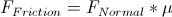### Example Question #1 : Friction And Normal Force

A crate of mass M is pushed along a frictionless horizontal floor by a force F acting downward from an angle xo above the horizontal. What is the magnitude of the normal force N between the crate and the floor?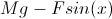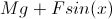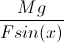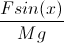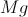Explanation:

Since the crate is not accelerating in the vertical direction, the sum of the vertical forces must be 0. In other words, the sum of the downwards forces must equal the sum of the upwards forces. In this case, the downwards forces on the crate are gravity (Mg) and the vertical component of the pushing force (Fsin(x)). The only force acting upwards is the normal force (N) from the floor. So, Mg + Fsin(x) = N. Note that the problem states the direction of the force to be from above the horizontal, meaning that the vertical component will add to the downward force.

### Example Question #1 : Friction And Normal Force

A 50kg block resting on the ground experiences an upward acceleration of 4m/s2. What is the normal force acting on the block?

700 N

300 N

200 N

500 N

300 N

Explanation:

The block experiences the force of gravity, plus the force of the upward acceleration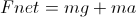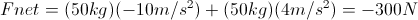If the block is resting on the ground, then its total force must be zero, and the normal force must cancel out the net force above. The normal force is 300N.

← Previous 1